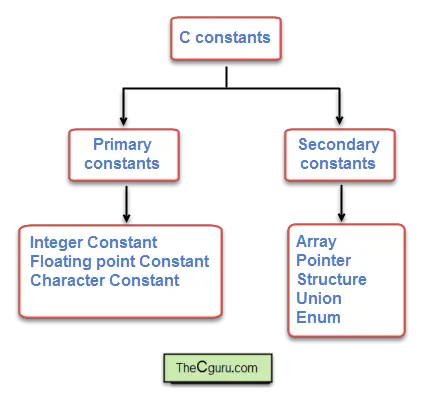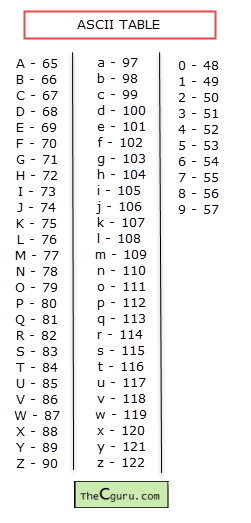# Constants in C

Last updated on July 27, 2020

A constant or sometimes called literal is an entity that doesn't change, whereas a variable is an entity that can change. In this section we will discuss constants, variables are discussed in lesson Variables in C.

## Types of constants #

In C there is two type of constants:

1. Primary constants.
2. Secondary constants.

Both types are further divided into more categories as shown in the figure.Here we will discuss only Primary constants, Secondary constants will be discussed later. To create Primary constants you need to obey the following rules.

## Numeric Constants #

Numeric constants are digits that may or may not have a decimal point. The rules for creating Numeric constants are:

1. Must have at least one digit.
2. No space, comma or any other special symbols are allowed.
3. It can be positive or negative if no sign precedes integer constant then it is assumed to be positive.

Numeric Constants are two types:

1. Integer constants
2. Floating point or real constants

### Integer constants #

Integer constants do not have decimal point (.). They can be written using decimal numbers (base 10), octal numbers(base 8) and hexadecimal (base 16).

Decimal constants

It contains digits between 0 and 9, but should not begin with a zero.

43, 199, 3452, -100


Octal constants

It contains digits between 0 and 7, and must begin with a zero.

012, 034, 01144


It contains digits from 0 to 9, and letters from a-f (either in uppercase or lowercase), and must always start with 0x or 0X.

0x23, 0Xff, 0x37a


As already said letters can be uppercase or lowercase.

0x23, 0XFF, 0x37A


We can also mix decimal, octal and hexadecimal.

int i = 12 + 045 + 0x3a


Always keep in mind that whatever number system we use to represent a number; they have no effect on how they are stored internally in memory (eventually every numbers and character are stored as streams of binary digits). Octal and hexadecimal are commonly used while writing low-level programs, so, in the beginning, you will not see them much.

By default, an integer constant is of type int. In a 32-bit system, int type occupies 4 bytes of memory. If the value of an integer is too large to fit into int then the type of the constant is promoted to long int. Again, if the value is still too large for the long int, then it will be promoted to unsigned long int eventually.

Rules for determining the type of octal and hexadecimal are slightly different and work as follows:

The compiler will go through the following type (top to bottom) until it finds a suitable type that can represent the constant.

 1 2 3 unsigned int long int unsigned long int 

You can also explicitly specify the type of an integer constant as long int by appending l or L:

10l, 3789L, 094l, 0xabL


Similarly, to specify the type of an integer constant as unsigned int append u or U after it:

23u, 034U, 0x8au


We can also use u or U and l or L to specify the type of an integer constant as an unsigned long int. 89ul - is of type unsigned long int

### Floating point or real constants #

Numeric constants having decimal point are called floating point or real constants. Floating-point constants can be written in two forms:

1. Fractional forms
2. Exponential form or Scientific notation

Here are the rules for creating floating point constants in Fractional form:

1. Must have one at least one digit
2. Must have a decimal point
3. Can be positive or negative, the default is positive
4. No comma, blanks, or any other symbols are allowed

Here are some examples:

 1 2 3 3.14 899.0 -0.999 

Exponential form is used in cases when a number is too small or too large. For example, 0.00000941 can be represented as 9.41e-6. The part of the number before e is called mantissa i.e 9.41, whereas, the part following e is called the exponent i.e -6.

Here are the rules for creating floating point constants in Exponential form:

1. Mantissa and exponent must be separated by e or E.
2. Mantissa can be positive or negative, default is positive.
3. Exponent must have at least one digit.
4. The exponent can be positive or negative default is positive

Some examples of floating point numbers in exponential form are:

 1 2 3 4 100.34e4 -56E10 0.233E10 -0.94e15 

By default, floating constants are of type double. We can explicitly mention the type of a floating-point constant as a float by appending f or F at the end of the constant. For example:

12f , -0.87f


Similarly, We can explicitly mention the type of a floating-point constant as long double by appending l or L at the end.

12.13l, -98.12L


## Character Constants #

A Character constant is a single alphabet, digit or any special symbol enclosed using single quotes. Here are some examples:

'A', 'c', '4', '\$', '^'


Note: Character constant must be always enclosed in single quotes, so the following is wrong.

 1 2 "a" "e" 

The maximum length of a character constant is 1 character long. That means you can not put more than one character inside single quotation marks like this:

 1 2 'ab' // Wrong '12' // Wrong 

As you may already know inside computer memory everything is stored as binary. It is easy to see how integer and floating point constants are stored as binary numbers. But how character constants are stored in the memory? It turns out that Every character constant has a unique integer associated with it. An ASCII table represents the decimal number used to represent every known character in the English Language.Consider the following statement:

char ch = 'a'; // declaring a variable ch and assigning 'a' to it


Here we are declaring a variable ch of type char and assigning a character constant 'a' to it (don't worry too much about the syntax right now, in the next chapter we will discuss variables in detail). Although it might appear that we are assigning 'a' to variable ch but actually what's gets assigned to ch is the ASCII value of 'a' i.e 97. Here is the little secret - In C, characters are treated as small integers, so they actually have int type rather than the char type. A char or signed char has range -127 to 128, while an unsigned char has range 0-255.

## String Constant #

String constants consist of zero or more characters enclosed in double quotes (""). At the end of the string, the null character i.e '\0' is automatically placed by the compiler. Here are some examples of string constants:

 1 2 3 "hello" "123" "" // This is empty string it consists of only one character '\0' which is added by the compiler automatically. 

Note: Although not officially part of Primary constants, string constants are given here for the sake of completeness. C has no data type for string, they are stored as an array of characters. We will learn more about strings in a great deal in its own chapter.

## Symbolic constants #

If we want to use constant several times in a program, then we can provide it a name. For example: If there is a need to use constant Π = 3.141592 at several places in the program, then we can give it a name and use that name instead of writing this long number. This constant is called Symbolic constant. It is generally defined at the beginning of the program. Here is the syntax of creating a Symbolic constant.

#define NAME VALUE


#define is a preprocessor directive just like #include, that's why it doesn't end with the semicolon (;).

NAME indicates the name we want to give to our constant and is generally written in uppercase.

VALUE can be numeric, character or string constant. Let's create a symbolic constant called PI.

#define PI 3.141592


When the program is compiled, the preprocessor replaces every occurrence of PI by its value. So that the statement:

printf("Circumference of circle = %f", 2*PI*4);


becomes

printf("Circumference of circle = %f", 2*3.141592*4);


Use of symbolic constant makes the program more maintainable and readable. For example: let's say we want more accurate results, so we decided to update the value of Π from 3.141592 to 3.14159265359, if we had not used symbolic constant then we would have to go through each occurrence of 3.141592 and update all of them. However, since we have already defined PI in a #define directive, we only need to make changes in the single place.